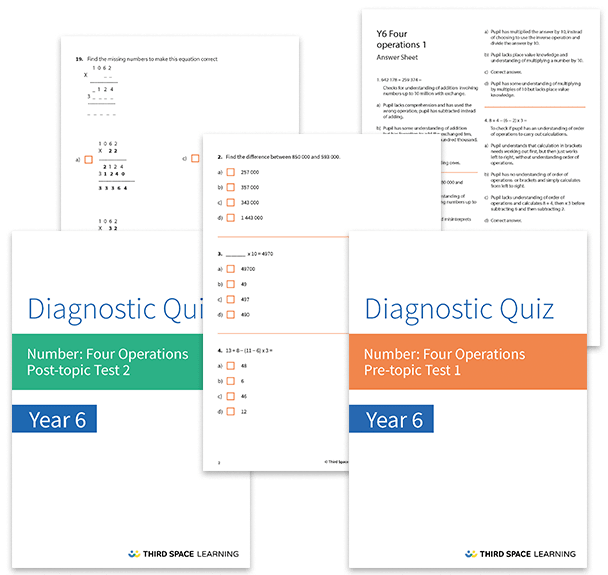# Four Operation Diagnostic Assessments Year 6

This pack of resources contains two diagnostic assessments for the four operations, one pre and one post topic assessment. Each test contains 25 multiple choice questions with detailed answers that not only indicate the correct answer but also explain the distractor answers. You can use these tests to help you diagnose specific misconceptions and learning gaps before starting a block of learning and after the block has been completed.

These tests cover:

• Understanding the order of operations
• Adding numbers to ten million
• Subtracting numbers to ten million
• Dividing three-digit numbers by 2-digit numbers (including interpreting remainders in context)
• Multiplying four-digit or three-digit numbers by two-digit numbers
• Identifying multiples and factors (including the lowest common multiple)
• Square, cube and prime numbers

Year 6

Multiplication, Addition, Division, Four operations, Subtraction